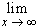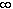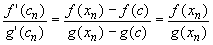#Interactive Real Analysis

Next | Previous | Glossary | Map

## 6.5. Differentiable Functions

### Theorem 6.5.15: l Hospital Rules

If f and g are differentiable in a neighborhood of x = c, and f(c) = g(c) = 0, thenprovided the limit on the right exists. The same result holds for one-sided limits.

If f and g are differentiable andf(x) =g(x) = -thenprovided the last limit exists.

### Proof:

The first part can be proved easily, if the right hand limit equals f'(c) / g'(c): Since f(c) = g(c) = 0 we have

•Taking the limit as x approaches c we get the first result. However, the actual result is somewhat more general, and we have to be slightly more careful. We will use a version of the Mean Value theorem:

Take any sequence {xn} converging to c from above. All assumptions of the generalized Mean Value theorem are satisfied (check !) on [c, xn]. Therefore, for each n there exists a number cn in the interval (c, xn) such that

•Taking the limit as n approaches infinity will give the desired result for right-handed limits. The proof is similar for left handed limits and therefore for 'full' limits.

The proof of the last part of this theorem is left as an exercise.Next | Previous | Glossary | Map